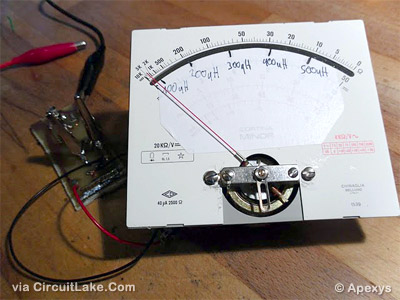# Circuit Lake

Electronic Project and Circuit Collection

## Inductivity Meter Using IC 555

07/02/2011 Category: Analog, Measurement, ProjectThe projects shows you how to build simple low cost Inductance Meter based on popular IC 555. The 555 works in its most simple rectangle oscillation configuration. In this mode, the duty-cycle should be around 50%, but this depends on the load on pin 3. Over the amplifying transistor Q1 the AC is given on an LC circuit. It oscillates a frequency given by the equation:These oscillating waves are amplified by Q2 and frequency is converted into a voltage by the RC-network of C3 and the amp meter. Due to the capacity of both C3 and C2 being constant, the current through the ampmeter is defined by the inductivity. The 555 inductance meter project is designed by Apexys. Download LTSpice File

L meter schematic